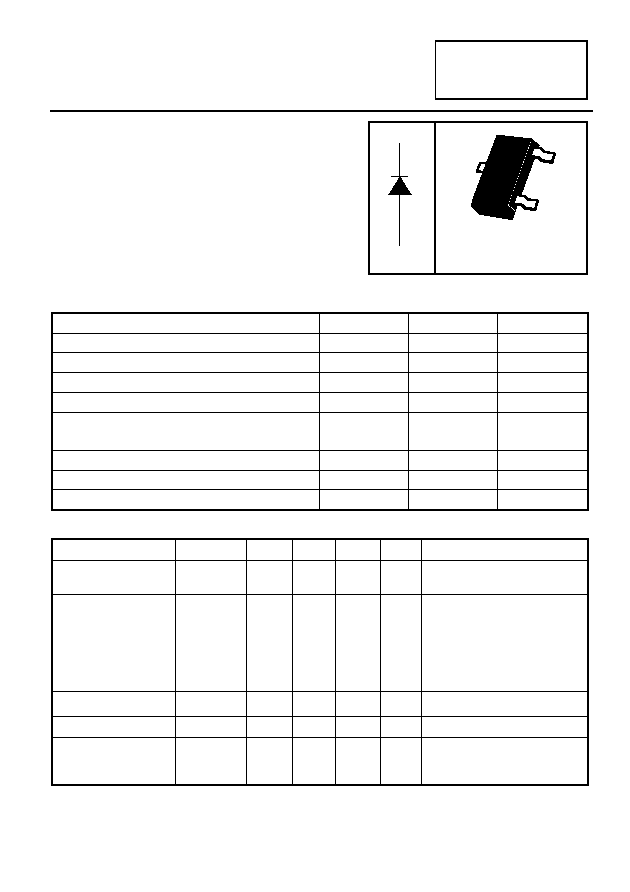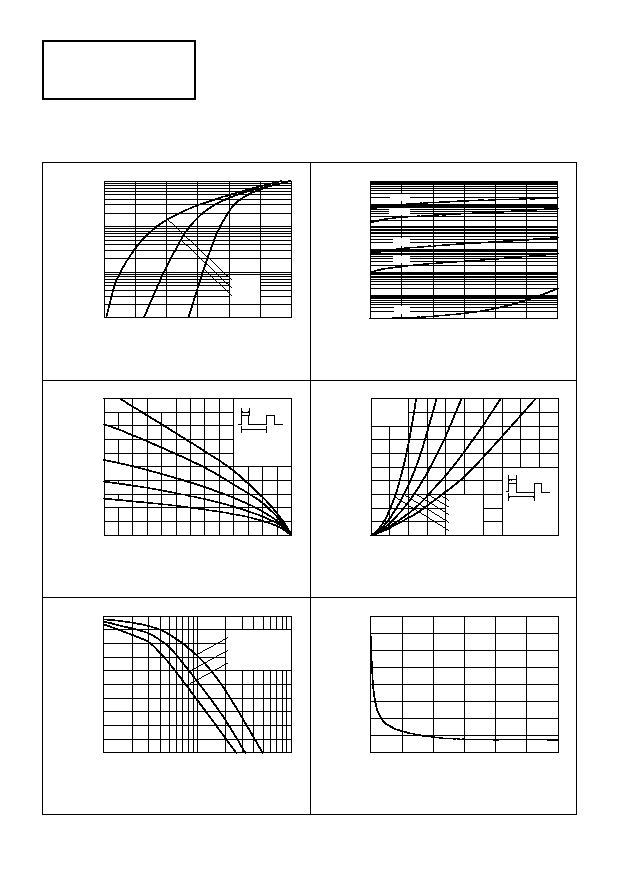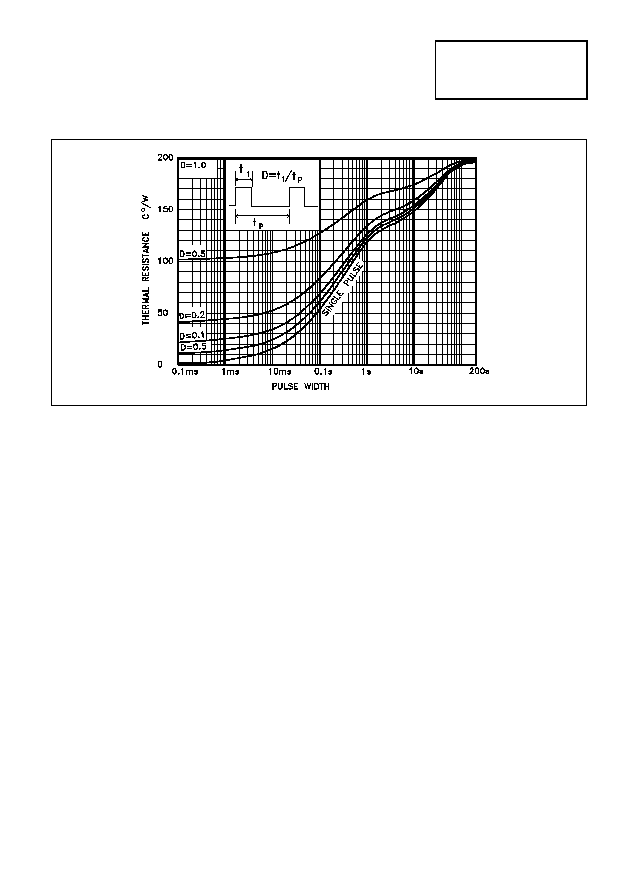–ü–ĺ–ł—Ā–ļ –ī–į—ā–į—ą–ł—ā–į (1.687.043 –ļ–ĺ–ľ–Ņ–ĺ–Ĺ–Ķ–Ĺ—ā–ĺ–≤) –ď–ī–Ķ –ł—Ā–ļ–į—ā—Ć–≤ –Ĺ–į–ł–ľ–Ķ–Ĺ–ĺ–≤–į–Ĺ–ł–ł–≤ –ĺ–Ņ–ł—Ā–į–Ĺ–ł–łDatasheet: ZHCS756 (Zetex Semiconductors)

Silicon High Current Schottky Barrier Diode –é¬įsuperbat–é¬Ī
–°–ļ–į—á–į—ā—Ć:PDFZIPSOT23 SILICON HIGH CURRENT
SCHOTTKY BARRIER DIODE "SuperBAT"
ISSUE 1- NOVEMBER 1997
7
FEATURES:
Low V
F
High Current Capability
APPLICATIONS:
DC - DC converters
Mobile telecomms
PCMCIA
PARTMARK DETAIL: S76
ABSOLUTE MAXIMUM RATINGS.
PARAMETER
SYMBOL
VALUE
UNIT
Continuous Reverse Voltage
V
R
60
V
Forward Current (Continuous)
I
F
750
mA
Forward Voltage @ I
F
= 750mA
V
F
610
mV
Average Peak Forward Current; D.C. = 50%
I
FAV
1500
mA
Non Repetitive Forward Current t
100
Ķ
s
t
10ms
I
FSM
12
5
A
A
Power Dissipation at T
amb
= 25į C
P
tot
500
mW
Storage Temperature Range
T
stg
-55 to + 150
į C
Junction Temperature
T
j
125
į C
ELECTRICAL CHARACTERISTICS (at T
amb
= 25į C unless otherwise stated).
PARAMETER
SYMBOL
MIN.
TYP.
MAX.
UNIT
CONDITIONS.
Reverse Breakdown
Voltage
V
(BR)R
60
80
V
I
R
= 300
Ķ
A
Forward Voltage
V
F
250
285
350
440
520
600
760
290
330
410
500
610
700
900
mV
mV
mV
mV
mV
mV
mV
I
F
= 50mA*
I
F
= 100mA*
I
F
= 250mA*
I
F
= 500mA*
I
F
= 750mA*
I
F
= 1000mA*
I
F
= 1500mA*
Reverse Current
I
R
50
100
Ķ
A
V
R
= 45V
Diode Capacitance
C
D
17
pF
f= 1MHz,V
R
= 25V
Reverse Recovery
Time
t
rr
12
ns
switched from
I
F
= 500mA to I
R
= 500mA
Measured at I
R
= 50mA
*Measured under pulsed conditions. Pulse width= 300
Ķ
s; duty cycle
2% .
ZHCS756
1
3
C
1
A
3
2
SOT23ZHCS756
0
60
1
10
100
0
60
0
0
60
V
F
- Forward Voltage (V)
I
F
v V
F
1m
I
F
- Forw
a
r
d
C
urrent (A)
I
F(a
v)
-

A
vg
F
w
d C
ur (A)
0
TC - Case Temperature ( C)
I
F(av)
v T
C
T
a
- Am
bient
T
emp
(įC
)
125
75
V
R
- Reverse Voltage (V)
T
a
v V
R
I
R
-
R
e
verse
C
urrent (A)
100n
V
R
- Reverse Voltage (V)
I
R
v V
R
P
F(a
v
)
-
A
vg
Pwr
Di
ss
(W)
0
I
F(av)
- Avg Fwd Curr (A)
P
F(av)
v I
F(av)
C
D
- Dio
de C
apacitance (pF)
160
80
0
V
R
- Reverse Voltage (V)
C
D
v V
R
-55įC
20
40
+25įC
+125įC
20
40
DC
D=0.5
D=0.2
D=0.1
D=0.05
DC
D=0.5
D=0.2
D=0.1
D=0.05
100
p
t
1
t
1
D=t
/t
p
I
F(pk)
I
F(av)
=DxI
F(pk)
Typical
Typical
Tj=125 C
F(av)
P
F(av)
=I
xV
F
0.1
0.2
0.3
0.4
0.5
0.6
1
P
I
t
1
F(pk)
=DxI
F(av)
=I
F(av)
F(av)
F
xV
F(pk)
p
t
/t
D=t
1
I
p
Rth=100įC/W
Rth=200įC/W
Rth=300įC/W
1m
100m
10m
1u
10u
100u
1m
10m
100m
0.2
0.4
0.6
0.8
1
70
80
90
100
110
120
0.1
0.2
0.3
0.4
0.5
0.2
0.4
0.6
0.8
1
+50įC
+25įC
+100įC
+125įC
-55įC
TYPICAL CHARACTERISTICSTYPICAL CHARACTERISTICS
ZHCS756
* Reference above figure, devices were mounted on a 15mmx15mm ceramic substrate.
MAXIMUM TRANSIENT THERMAL RESISTANCE